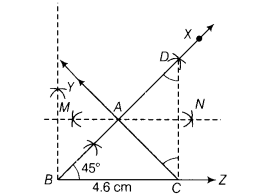# Construct a ∆ABC in which BC = 4.6 cm, ∠B =45° and AB + CA =8.2 cm

Construct a ∆ABC in which BC = 4.6 cm, ∠B =45° and AB + CA =8.2 cm.

Given, BC = 4.6 cm, ∠B = 45“ and AB + CA = 8.2 cm
In horizontal axis take 1 block is equal to 10 units and in vertical axis, take 1 block is equal to 5 units.
Steps of construction
(i) Draw the base line segment BC = 4.6 cm.
(ii) At the point S, make ∠XBC = 45“.
(iii) Now, cut a line segment BD equal to AB + AC = 8.2 cm from the ray BX.
(iv) Join DC.(v)Draw perpendicular bisector MN of CD which meets BX at A.
(vi)On joining AC, we get the required ∆ABC